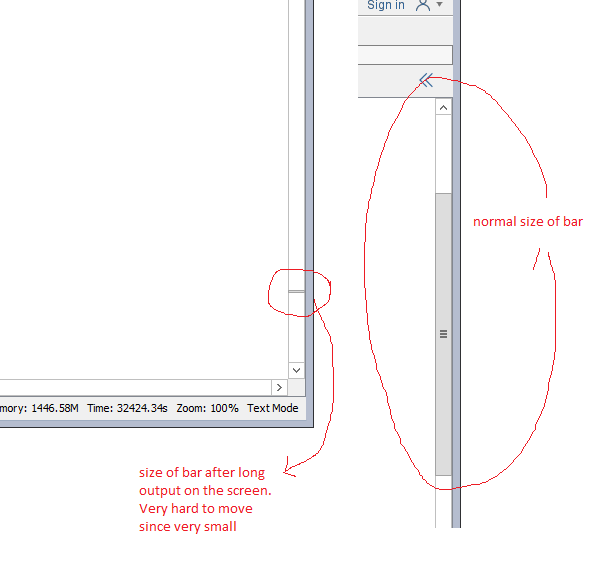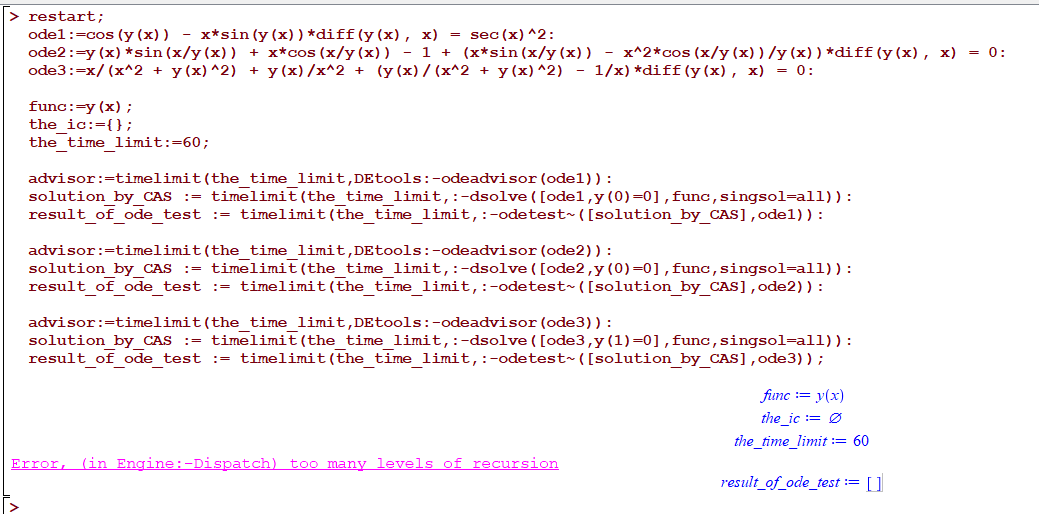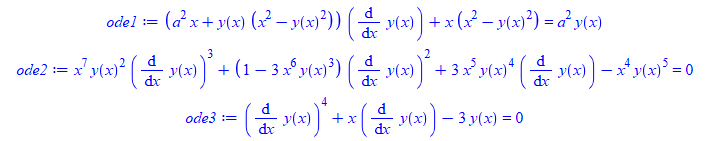## Conversion of Maple code to MATLAB...

Hello

I want to know if there is any option to convert the Maple code into MATLAB version to work across platforms. I have tried the code generation option in Maple and I could not understand how to use the single line code generated. I am working on an already written Maple code and conversion of code to use on both platforms is kind of important. Please advice me on the same.

Regards!

## How to draw phase portrait?...

I'm willing to draw phase portrait for the equation of ode fifth order

u'''''+6(u'u'''+(u'')^2)-u'''(a+2b)=0

where a and b are arbitrary constant not equal to zero and (') represents derivative w.r.t  'x'.

## Multiuser on Maple Workbook...

We have just run into a problem (loss of data), when 2 users have opened the same workbook.

Does Maple support Multiuser access to a workbook, and if not - why isn't there any lock mechanism in place to prevent that?

## how to enable autoscrolling in worksheet mode?...

https://www.mapleprimes.com/questions/203628-Maple-2015-No-Longer-Autoscroll-As

And according to the reply there at the time, this is supposed to have been fixed in Maple 2015.1:

It appears that the scrolling issue has been fixed in the next version of Maple

But ever since then, whenever I use Maple, the worksheet do not auto-scroll as the program runs and prints outout to the screen. I have to keep using the mouse to move the bar down to see the latest prints.

Not only that, the bar itself (on the right side) shrinks in size as more outpout is printed.

It becomes smaller and smaller with time, and becomes very hard to grab it with the mouse since it become so thin.  Once all the output is deleted, the bar become long again.

So I think there is an option to enable autoscrolling somewhere.

But I am not able to find it. I looked at options, display, general and interface and see nothing there to turn it on.

Sometimes I get the feeling that the folks at Maplesoft do not use Maple interface the way we users use it, becuase this is such a basic interface problem and any one who uses Maple interface for more than one day must see these problems all the time.

So how come these are still not fixed?  Here is a screen shot showing the bar on the right after long print out, and showing how it was before.I use only worksheet mode, not document mode. On windows 10.

## Get eigenvectors in Maple ...

Hi, I want to use Maple to derive the eigenvectors of a 3x3 matrix A. I can obtian the 3 eigenvalues correctly by using eigenvalues(A), and the results are "u, -a+u, a+u". But after inputing eigenvectors(A), I can only get "[-a+u, 1, {r}], [a+u, 1, {r}], [u, 1, {r}]". All the 3 eigenvectors are written as {r}, I dont know why...and how can I tranform {r} into visiable results?

I would be very appreciate if anyone could give me some help!

## orthogonal affinity to oblique line and k ratio...

How to draw a circle transfomed by orthogonal affinity to oblique line and k ration ? Thank you.

## Simulate murmuration...

Help me please.  How can i simulate murmuration in maple.

## random error Error, (in Engine:-Dispatch) too many...

it took a while to track this. When I run these 3 ode's in this sequence, Maple gives

Error, (in Engine:-Dispatch) too many levels of recursion

and it has to be this sequence.  I also found I might have to run the code 2 or 3 times to get this error if it does not show first time.

Will show a movie below also.

Here is the code

```restart;
ode1:=cos(y(x)) - x*sin(y(x))*diff(y(x), x) = sec(x)^2:
ode2:=y(x)*sin(x/y(x)) + x*cos(x/y(x)) - 1 + (x*sin(x/y(x)) - x^2*cos(x/y(x))/y(x))*diff(y(x), x) = 0:
ode3:=x/(x^2 + y(x)^2) + y(x)/x^2 + (y(x)/(x^2 + y(x)^2) - 1/x)*diff(y(x), x) = 0:

func:=y(x);
the_ic:={};
the_time_limit:=60;

solution_by_CAS := timelimit(the_time_limit,:-dsolve([ode1,y(0)=0],func,singsol=all)):
result_of_ode_test := timelimit(the_time_limit,:-odetest~([solution_by_CAS],ode1)):

solution_by_CAS := timelimit(the_time_limit,:-dsolve([ode2,y(0)=0],func,singsol=all)):
result_of_ode_test := timelimit(the_time_limit,:-odetest~([solution_by_CAS],ode2)):

solution_by_CAS := timelimit(the_time_limit,:-dsolve([ode3,y(1)=0],func,singsol=all)):
result_of_ode_test := timelimit(the_time_limit,:-odetest~([solution_by_CAS],ode3));
```And here is a movie (this time it happend on second run) sometimes it happens on first run. so it is random.Maple 2020.1

Why does it happen and is there a work around?

## few errors using dsolve and and odetest...

Noticed few errors generated on some ode's. I do not know if these are expected or known, I thought may be someone might want to check them.

```restart;
ode1:=(a^2*x+y(x)*(x^2-y(x)^2))*diff(y(x),x)+x*(x^2-y(x)^2) = a^2*y(x);
ode2:=x^7*y(x)^2*diff(y(x),x)^3+(1-3*x^6*y(x)^3)*diff(y(x),x)^2+3*x^5*y(x)^4*diff(y(x),x)-x^4*y(x)^5 = 0;
ode3:=diff(y(x),x)^4+x*diff(y(x),x)-3*y(x) = 0;
````sol:=timelimit(60,:-dsolve(ode1,y(x))):`

Error, (in dsolve) numeric exception: division by zero

```sol:=timelimit(60,:-dsolve(ode2,y(x))):
timelimit(60,:-odetest([sol],ode2))
```

Error, (in IntegrationTools:-Indefinite:-AlgebraicFunction) argument is not an algebraic

```sol:=timelimit(60,:-dsolve(ode3,y(x))):
timelimit(60,:-odetest(sol,ode3));
timelimit(60,:-odetest(sol,ode3))
```

Error, (in odetest) received not an ODE w.r.t x(_T)
Error, (in odetest) received not an ODE w.r.t y(_T)

This last one is only due to how the solution is given. So I do not think it is a real problem.

Maple 2020.1, Physics 708

windows 10

Maple Worksheet - Error
Failed to load the worksheet /maplenet/convert/check.mw .

## Does any one having the codes to compute Lie brack...

I'm searching for the codes in maple to calculate the Lie bracket and adjoint actions on the infinitesimal vectors.

For eg. if I'm having my vector V3 and V4 as## How do I solved PDE using non classical numerical ...

Dear All.

I hope we are all staying safe.

Please I want to solve Sine Gordon Equation using a numerical method I constructed (non-classical), I need to compare the result of the method with the exact solution to generate the errors. However, since the exact solution has two variables, x, and t, I really don't know how to accommodate the two in my coding.

Can someone be of help in this regard?

Thank you and kind regards

## Why inequal plot like this?...

Hi there,

Can someone explain to me why is it happening? If smaller equal than coloured well, if smaller then just the equal line appears. Why?

```with(plots);
dm1 := .23; pm12 := .7; dm12 := .23;
inequal(pm1*(dm12+am21)-pm12*(dm1+am21) <= 0, pm1 = 0 .. 1, am21 = 0 .. 1, color = "Nautical 1");

````inequal(pm1*(dm12+am21)-pm12*(dm1+am21) < 0, pm1 = 0 .. 1, am21 = 0 .. 1, color = "Nautical 1")`Thank you!

## Ellipse Equation ( rotation)...

Hi ,

i want to find simple equation of rotate Ellipse  ( El1,EL2,El3 in the  worksheet), with calssic formulaIdeas? Thanks

QuestionConiqueRotation.mw

## Find the focus of an ellipse with a tangential gen...

Etude d'un cas particulier a := 5: b := 7: k := 9: A := [a, 0]: B := [0, b]: #A et B fixes P := [t, 0]: Q := [0, k/t]:#P et Q 2 points mobiles cir := -a*x-b*y+x^2+y^2 = 0: sol := solve(subs(y = 5, cir), x): cen := [solve(diff(cir, x)), solve(diff(cir, y))]: x0 := sol: y0 := 5: M := [x0, y0]: R := sqrt(cen^2+cen^2): beta := arctan(diff(solve(EQ(M, cen), y), x)): Recherche des valeurs de t pour que les 2 droites soient perpendiculaires eq := t^2*(y0-b)+t*(a*b-a*y0+b*x0-k)-x0*(a*b-k) = 0; sol := solve(eq, t); t := sol; tp := sol; P1 := [t, 0]; Q1 := [0, k/t]; PQ1 := simplify(x*(-a*b+b*t+k)+y*t*(t-a)-t*(-a*b+b*t+k)) = 0:#1ere tangente PQ2 := simplify(x*(-a*b+b*tp+k)+y*tp*(tp-a)-tp*(-a*b+b*tp+k)) = 0:#2ième tangente P2 := [tp, 0]; Q2 := [0, k/tp]; CIR := implicitplot(cir, x = -4 .. 8, y = -4 .. 12, color = red); Fig := proc (alpha) local Dr1, DR1, Dr2, DR2, N, u0, v0, Po, t, tp, sol; global a, b, k, cen, R; u0 := cen+R*cos(alpha); v0 := cen+R*sin(alpha); N := [u0, v0]; sol := solve(t^2*(v0-b)+t*(b*u0-a*v0+a*b-k)-u0*(a*b-k) = 0, t); t := sol; tp := sol; Dr1 := simplify(x*(-a*b+b*t+k)+y*t*(t-a)-t*(-a*b+b*t+k)) = 0; DR1 := implicitplot(Dr1, x = -4 .. 8, y = -4 .. 12, color = brown); Dr2 := simplify(x*(-a*b+b*tp+k)+y*tp*(tp-a)-tp*(-a*b+b*tp+k)) = 0; DR2 := implicitplot(Dr2, x = -4 .. 8, y = -4 .. 12, color = pink); Po := pointplot([N[]], symbol = solidcircle, color = [black], symbolsize = 8); display([Po, DR1, DR2]) end proc; DrPQ1 := implicitplot(PQ1, x = -4 .. 22, y = -4 .. 12, color = blue); DrPQ2 := implicitplot(PQ2, x = -4 .. 22, y = -4 .. 12, color = blue); Points := pointplot([A[], B[], M[], P1[], P2[], Q1[], Q2[], cen[]], symbol = solidcircle, color = [green], symbolsize = 10); T := plots:-textplot([[A[], "A"], [B[], "B"], [M[], "M"], [P1[], "P1"], [P2[], "P2"], [Q1[], "Q1"], [Q2[], "Q2"], [cen[], "cen"]], font = [times, 10], align = {below, left}); n := 19; display([seq(Fig(2*i*Pi/n), i = 0 .. n), Fig(beta), CIR, DrPQ1, DrPQ2, Points, T], scaling = constrained, size = [500, 500]); I would find out the focus of the ellipse. Thank you.

## Homotopy Analysis Method, NOPH package and solutio...

Hello, dear forum users!

Does anyone use the method of homotopy analysis (HAM) and the NOPH package in their work. (moderator: link)It seems to me that only HAM can help.